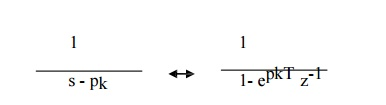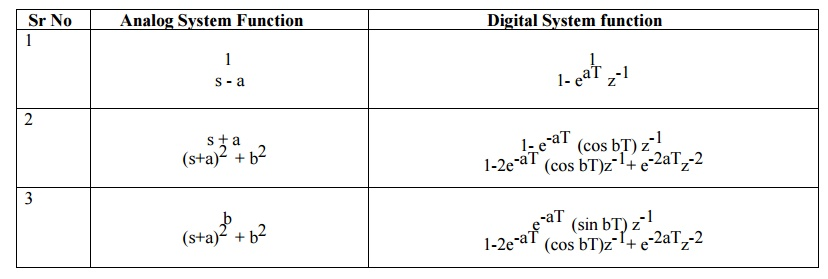Home | | Discrete Time Systems and Signal Processing | Conversion of Analog Filter into Digital Filter

# Conversion of Analog Filter into Digital Filter

The unit sample response of the digital filter is obtained by uniform sampling of ha(t). h(n) = ha(nT)

CONVERSION OF ANALOG FILTER INTO DIGITAL FILTER

Let the system function of analog filter is n

Ha(s)= ΣK+1 Ck / s-pk (1) k=1

where pk are the poles of the analog filter and ck are the coefficients of partial fraction expansion. The impulse response of the analog filter ha(t) is obtained by inverse Laplace transform and given asThe unit sample response of the digital filter is obtained by uniform sampling of ha(t). h(n) = ha(nT)

n=0,1,2. ………….System function of digital filter H(z) is obtained by Z transform of h(n).Using the standard relation and comparing equation (1) and (4) system function of digital filter is given asSTANDARD RELATIONS IN IIR DESIGNEXAMPLES - IMPULSE INVARIANCE METHODStudy Material, Lecturing Notes, Assignment, Reference, Wiki description explanation, brief detail
Digital Signal Processing : IIR Filter Design : Conversion of Analog Filter into Digital Filter |